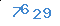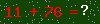• 10+
机构授权
• 180+
国家/地区
• 全球域名

• 全球域名可供申请
• 域名极速解析
• 域名过期赎回
• 域名回购谈判
• 代提供当地资料
• 一对一跟踪服务
• 保姆式更新维护

• .com
• .net
• .org
• .gov
• .edu
• .mobi
• .info
• .biz
• .tm
• .tv
• .asia
• .me
• .travel
• .tel
• .neme
• .top
• .pro
• .mil
• .arts
• .firm
• .int
• .nom
• .rec
• .store
• .museum
• .coop
• .aero
• .xxx
• .idv
• .ae
• .af
• .ag
• .ai
• .al
• .am
• .an
• .ao
• .aq
• .ar
• .as
• .at
• .au
• .aw
• .az
• .ba
• .bb
• .bd
• .be
• .bf
• .bg
• .bh
• .bi
• .bj
• .bm
• .bn
• .bo
• .br
• .bs
• .bt
• .bv
• .by
• .bz
• .ca
• .cc
• .cf
• .cg
• .ch
• .ci
• .ck
• .cl
• .cm
• .cn
• .co
• .cq
• .cr
• .cu
• .cv
• .cx
• .cy
• .cz
• .de
• .dj
• .dk
• .dm
• .do
• .dz
• .ec
• .ee
• .eg
• .eh
• .es
• .et
• .ev
• .fi
• .fj
• .fk
• .fm
• .fo
• .fr
• .ga
• .gb
• .gd
• .ge
• .gf
• .gh
• .gi
• .gl
• .gm
• .gn
• .gp
• .gr
• .gt
• .gu
• .gw
• .gy
• .hk
• .hm
• .hn
• .hr
• .ht
• .hu
• .id
• .ie
• .il
• .in
• .io
• .iq
• .ir
• .is
• .it
• .jm
• .jo
• .jp
• .ke
• .kg
• .kh
• .ki
• .km
• .kn
• .kp
• .kr
• .kw
• .ky
• .kz
• .la
• .lb
• .lc
• .li
• .lk
• .lr
• .ls
• .lt
• .lu
• .lv
• .ly
• .ma
• .mc
• .md
• .mg
• .mh
• .mm
• .mn
• .mo
• .mp
• .mq
• .mr
• .ms
• .mt
• .mv
• .mw
• .mx
• .my
• .mz
• .na
• .nc
• .ne
• .nf
• .ng
• .ni
• .nl
• .no
• .np
• .nr
• .nt
• .nu
• .nz
• .om
• .qa
• .pa
• .pe
• .pf
• .pg
• .ph
• .pk
• .pl
• .pm
• .pn
• .pr
• .pt
• .pw
• .py
• .re
• .ro
• .ru
• .rw
• .sa
• .sb
• .sc
• .sd
• .se
• .sg
• .sh
• .si
• .sj
• .sk
• .sl
• .sm
• .sn
• .so
• .sr
• .st
• .su
• .sy
• .sz
• .tc
• .td
• .tf
• .tg
• .th
• .tj
• .tk
• .tm
• .tn
• .to
• .tp
• .tr
• .tt
• .tv
• .tw
• .tz
• .ua
• .ug
• .uk
• .us
• .uy
• .va
• .vc
• .ve
• .vg
• .vn
• .vu
• .wf
• .ws
• .ye
• .yu
• .za
• .zm
• .zr
• .zw
• .中国
• .网络
• .公司
• .商标
• .商城
• .商店
• .网店
• .网址
• .集团
• .信息
• .在线
• .中文网
• .手机
• .广东
• .佛山
• .企业
• .游戏
• .娱乐
• .我爱你
• .com
• .cn
• .co
• .net
• .biz
• .auto
• .wang
• .xyz
• .shop
• .手机
• .商标
• .集团
• .商城
• .网址
• .游戏
• .娱乐
• .中文网
• .商店
• .我爱你
• .广东
• .佛山
• .企业
• .在线
• .网店
• .club
• .wiki
• .xin
• .love
• .tech
• .online
• .shop
• .xyz
• .wang
• .ltd
• .group
• .top
• .fun
• .store
• .ltd
• .red
• .kim
• .work
• .vip
• .cool
• .chat
• .world
• .city
• .company
• .today
• .team
• .video.
• .guru
• .run
• .email
• .life
• .market
• .pub
• .sale
• .social
• .studio
• .auto
• .site
• .ink
• .zone
• .show
• .center
• .fund
• .gold
• .plus
• .design
• .art
• .live
• .beer
• .ren
• .gov.cn
• .ac.cn
• .bj.cn
• .sh.cn
• .tj.cn
• .ln.cn
• .jl.cn
• .hl.cn
• .js.cn
• .sd.cn
• .ha.cn
• .hb.cn
• .hn.cn
• .gz.cn
• .yn.cn
• .xz.cn
• .sn.cn
• .tw.cn
• .hk.cn
• .mo.cn
• .cq.cn
• .he.cn
• .sx.cn
• .nm.cn
• .zj.cn
• .ah.cn
• .fj.cn
• .jx.cn
• .gd.cn
• .gx.cn
• .hi.cn
• .se.cn
• .gs.cn
• .qh.cn
• .nx.cn
• .xj.cn扫一扫 关注易网